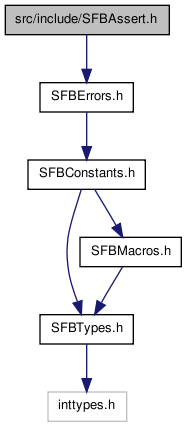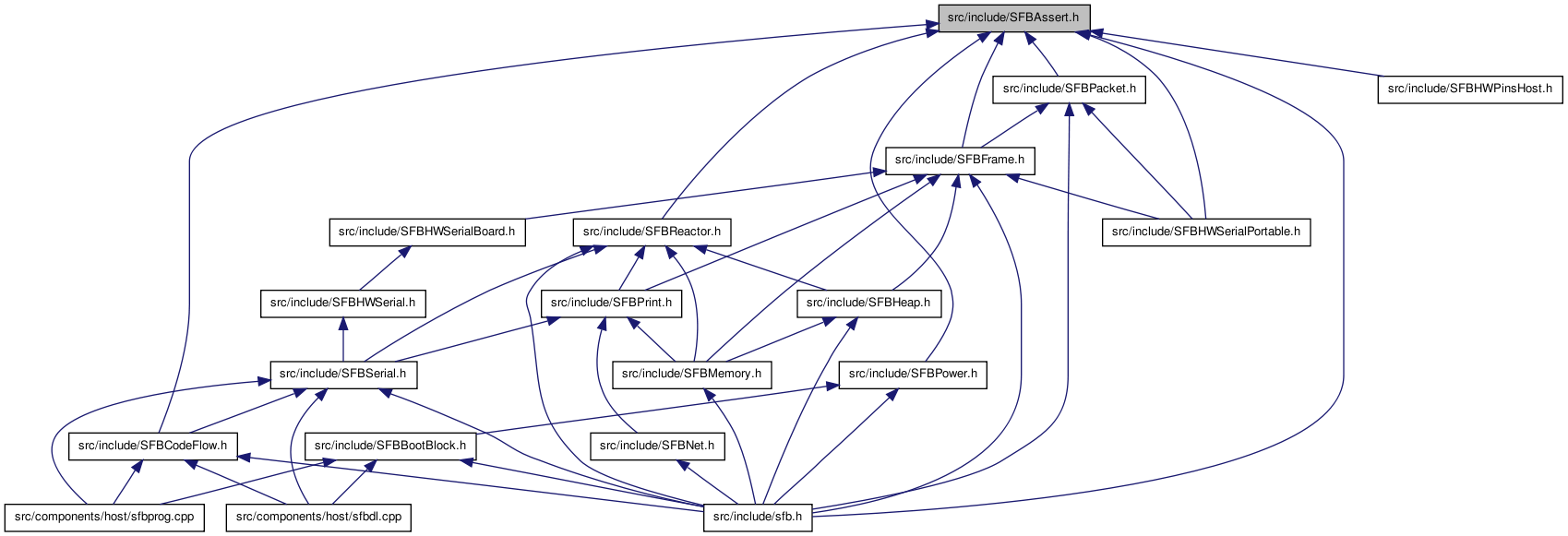# SFBAssert.h File Reference

Support for assertions. More...

`#include "SFBErrors.h"`

Include dependency graph for SFBAssert.h:This graph shows which files directly or indirectly include this file:Go to the source code of this file.

## Defines

#define API_BUG(code)   do { _apiError_(code,__FILE__,__LINE__); } while (0)
Causes an SFB to die immediately, blinking the given code.
#define API_ASSERT(condition, code)   do { if (!(condition)) API_BUG(code); } while (0)
Causes an SFB to die if condition is false, blinking the given code.
Causes the sketch to die immediately with a 'POISON'-related blinkcode.
#define API_ASSERT_MAX_CODE(value, maxval, code)   API_ASSERT(((u32)(value))<(maxval),code)
Assert that value is in the range 0.
#define API_ASSERT_MAX(value, maxval)   API_ASSERT_MAX_CODE(value,maxval,E_API_MAX_RANGE)
Assert that value is in the range 0.
#define API_ASSERT_TRUE(value)   API_ASSERT((value)!=0,E_API_TRUE)
Assert that value is non-zero, else die.
#define API_ASSERT_FALSE(value)   API_ASSERT((value)==0,E_API_FALSE)
Assert that value is zero, else die.
#define API_ASSERT_NONZERO(value)   API_ASSERT((value)!=0,E_API_NONZERO)
Assert that value is non-zero, else die.
#define API_ASSERT_NONNULL(value)   API_ASSERT((value)!=0,E_API_NULL_POINTER)
Assert that value is non-zero, else die.
#define API_ASSERT_NULL(value)   API_ASSERT((value)==0,E_API_NONNULL_POINTER)
Assert that value is zero, else die.
#define API_ASSERT_ZERO(value)   API_ASSERT((value)==0,E_API_ZERO)
Assert that value is zero, else die.
#define API_ASSERT_EQUAL(target, value)   API_ASSERT((value)==(target),E_API_EQUAL)
Assert that target is equal to value, else die.
#define API_ASSERT_NOT_EQUAL(target, value)   API_ASSERT((value)!=(target),E_API_NOT_EQUAL)
Assert that target is not equal to value, else die.
#define API_ASSERT_LESS(target, value)   API_ASSERT((target)<(value),E_API_LESS)
Assert that target is less than to value, else die.
#define API_ASSERT_LESS_EQUAL(target, value)   API_ASSERT((target)<=(value),E_API_LESS_EQUAL)
Assert that target is less than or equal to value, else die.
#define API_ASSERT_GREATER(target, value)   API_ASSERT((target)>(value),E_API_GREATER)
Assert that target is greater than value, else die.
#define API_ASSERT_GREATER_EQUAL(target, value)   API_ASSERT((target)>=(value),E_API_GREATER_EQUAL)
Assert that target is greater than or equal to value, else die.
Assert that u32Pin is a valid pin number, else die.
Assert that u32Face is a valid 'physical face' number, else die.
Assert that u32Face is a valid face number in the loosest possible sense, else die.
Assert that u32address is an even number, else die.
Assert that u32address is a multiple of four, else die.
Assert that u32address is a multiple of eight, else die.

## Detailed Description

Support for assertions.

Date:
The GNU Lesser General Public License
All code samples shown in documentation are placed into the public domain.

## Define Documentation

 #define API_ASSERT ( condition, code ) do { if (!(condition)) API_BUG(code); } while (0)

Causes an SFB to die if condition is false, blinking the given code.

Example:
```   ...
API_ASSERT(foo < bar,E_BUG_INCONSISTENT_STATE);
...
void function(int arg) {
API_ASSERT(arg >= -1 && arg < 256,E_API_ARGUMENT);
...
```

Assert that u32address is a multiple of eight, else die.

E_API_DOUBLEWORD_ALIGNED

 #define API_ASSERT_EQUAL ( target, value ) API_ASSERT((value)==(target),E_API_EQUAL)

Assert that target is equal to value, else die.

E_API_EQUAL

 #define API_ASSERT_FALSE ( value ) API_ASSERT((value)==0,E_API_FALSE)

Assert that value is zero, else die.

E_API_FALSE

 #define API_ASSERT_GREATER ( target, value ) API_ASSERT((target)>(value),E_API_GREATER)

Assert that target is greater than value, else die.

E_API_GREATER

 #define API_ASSERT_GREATER_EQUAL ( target, value ) API_ASSERT((target)>=(value),E_API_GREATER_EQUAL)

Assert that target is greater than or equal to value, else die.

E_API_GREATER_EQUAL

Assert that u32address is an even number, else die.

E_API_HALFWORD_ALIGNED

 #define API_ASSERT_LESS ( target, value ) API_ASSERT((target)<(value),E_API_LESS)

Assert that target is less than to value, else die.

E_API_LESS

 #define API_ASSERT_LESS_EQUAL ( target, value ) API_ASSERT((target)<=(value),E_API_LESS_EQUAL)

Assert that target is less than or equal to value, else die.

E_API_LESS_EQUAL

 #define API_ASSERT_MAX ( value, maxval ) API_ASSERT_MAX_CODE(value,maxval,E_API_MAX_RANGE)

Assert that value is in the range 0.

.maxval-1, else die.

E_API_MAX_RANGE

 #define API_ASSERT_MAX_CODE ( value, maxval, code ) API_ASSERT(((u32)(value))<(maxval),code)

Assert that value is in the range 0.

.maxval-1, else die with code.

the supplied code, as produced by the BLINK_MAP macro

 #define API_ASSERT_NONNULL ( value ) API_ASSERT((value)!=0,E_API_NULL_POINTER)

Assert that value is non-zero, else die.

E_API_NULL_POINTER
Examples:
zformat1.cpp, zformat2.cpp, and zformat3.cpp.

 #define API_ASSERT_NONZERO ( value ) API_ASSERT((value)!=0,E_API_NONZERO)

Assert that value is non-zero, else die.

E_API_NONZERO

 #define API_ASSERT_NOT_EQUAL ( target, value ) API_ASSERT((value)!=(target),E_API_NOT_EQUAL)

Assert that target is not equal to value, else die.

E_API_NOT_EQUAL

 #define API_ASSERT_NULL ( value ) API_ASSERT((value)==0,E_API_NONNULL_POINTER)

Assert that value is zero, else die.

E_API_NONNULL_POINTER

 #define API_ASSERT_TRUE ( value ) API_ASSERT((value)!=0,E_API_TRUE)

Assert that value is non-zero, else die.

E_API_TRUE

 #define API_ASSERT_VALID_EXTENDED_FACE ( u32Face ) API_ASSERT_MAX_CODE(u32Face,MAX_FACE_INDEX,E_API_BAD_FACE)

Assert that u32Face is a valid face number in the loosest possible sense, else die.

Note there are several different notions of 'face' used in different contexts; this assertion is the most liberal, allowing the physical faces NORTH, SOUTH, EAST, and WEST, as well as 'internal' faces such as SPINE and BRAIN, and potential 'virtual' faces defined by sketch code. Compare to API_ASSERT_VALID_FACE.

 #define API_ASSERT_VALID_FACE ( u32Face ) API_ASSERT_MAX_CODE(u32Face,FACE_COUNT,E_API_BAD_FACE)

Assert that u32Face is a valid 'physical face' number, else die.

Note there are several different notions of 'face' used in different contexts; this assertion, allowing only the physical faces NORTH, SOUTH, EAST, and WEST, is the most restrictive. Compare to API_ASSERT_VALID_EXTENDED_FACE.

 #define API_ASSERT_VALID_PIN ( u32Pin ) API_ASSERT_MAX_CODE(u32Pin,VIRTUAL_PIN_COUNT,E_API_BAD_PIN)

Assert that u32Pin is a valid pin number, else die.

Assert that u32address is a multiple of four, else die.

E_API_WORD_ALIGNED

 #define API_ASSERT_ZERO ( value ) API_ASSERT((value)==0,E_API_ZERO)

Assert that value is zero, else die.

E_API_ZERO

 #define API_BUG ( code ) do { _apiError_(code,__FILE__,__LINE__); } while (0)

Causes an SFB to die immediately, blinking the given code.

Example:
```   ...
if (foo < bar) API_BUG(E_BUG_INCONSISTENT_STATE);
...
switch (ch) {
default: API_BUG(BLINK_MAP(0,0,1);  // Die with custom code (1 blue only)
...
```

 #define POISON ( code0_6 ) API_BUG(BLINK_MAP(1,0,code0_6))

Causes the sketch to die immediately with a 'POISON'-related blinkcode.

Used for basic debugging, to determine when execution has reached a particular point in the code. The argument, a number from 0 to 6, determines which code is 'blinked'; this can be used to distinguish multiple possible poisoning locations.

```     void myHandler(u8 *) {
POISON(0);  // XXX IS MY HANDLER EVEN GETTING CALLED?
..stuff..
}
```
E_API_DEBUG_POISON0 if `POISON(0)` is executed
E_API_DEBUG_POISON1 if `POISON(1)` is executed
E_API_DEBUG_POISON2 if `POISON(2)` is executed
E_API_DEBUG_POISON3 if `POISON(3)` is executed
E_API_DEBUG_POISON4 if `POISON(4)` is executed
E_API_DEBUG_POISON5 if `POISON(5)` is executed
E_API_DEBUG_POISON6 if `POISON(6)` is executed